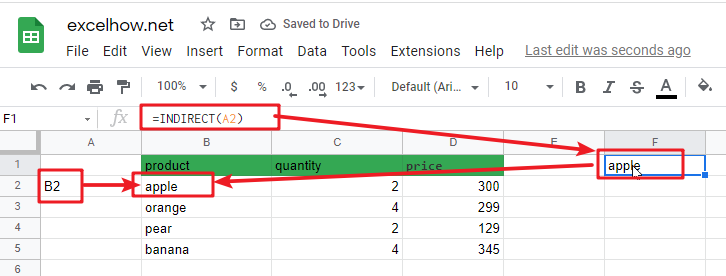# ExcelHow

This post will guide you how to use Google Sheets INDIRECT function with syntax and examples.

## Description

The Google Sheets INDIRECT function  returns the cell reference based on a text string, such as: type the text string “A2” in B1 cell, it just a text string, so you can use INDIRECT function to convert text string as cell reference.

The INDIRECT function can be used to get a valid cell reference from a given text string in google sheets. The purpose of this function is to get a cell reference from a text string, and the returned value is a valid cell reference.

The INDIRECT function is a build-in function in Google Sheets and it is categorized as a Lookup function.

## Syntax

The syntax of the INDIRECT function is as below:

= INDIRECT(ref_text,[a1])

Where the INDIRECT function arguments are:

• Ref_text -This is a required argument. A reference to a cell as a text string.
• A1 – This is an optional argument. It is a logical value that specify the style of the Ref_text reference.

Note:

• If A1 value is True or omitted, the ref_text will be interpreted as an A1-style reference.
• If A1 value is False, the ref_text will be interpreted as R1C1 style reference.

## Google Sheets INDIRECT Function Examples

The below examples will show you how to use google sheets INDIRECT Function to return a  cell or range reference that is represented by a text string.

#1 To get the value of the reference in cell A2, just using the following INDIRECT formula:

`=INDIRECT(A2)`